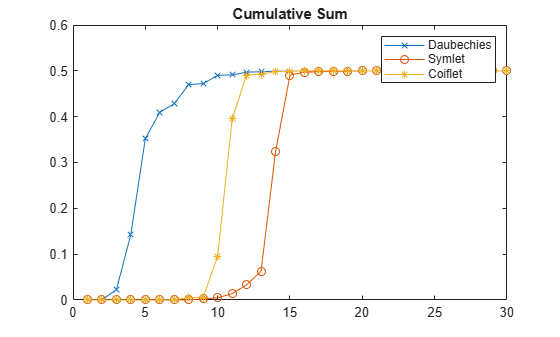# Extremal Phase

This example demonstrates that for a given support, the cumulative sum of the squared coefficients of a scaling filter increase more rapidly for an extremal phase wavelet than other wavelets.

Generate the scaling filter coefficients for the `db15` and `sym15` wavelets. Both wavelets have support of width $2×15-1=29$.

```[~,~,LoR_db,~] = wfilters('db15'); [~,~,LoR_sym,~] = wfilters('sym15');```

Next, generate the scaling filter coefficients for the `coif5` wavelet. This wavelet also has support of width $6×5-1=29$.

`[~,~,LoR_coif,~] = wfilters('coif5');`

Confirm the sum of the coefficients for all three wavelets equals $\sqrt{2}$.

`sqrt(2)-sum(LoR_db)`
```ans = 2.2204e-16 ```
`sqrt(2)-sum(LoR_sym)`
```ans = 0 ```
`sqrt(2)-sum(LoR_coif)`
```ans = 2.2204e-16 ```

Plot the cumulative sums of the squared coefficients. Note how rapidly the Daubechies sum increases. This is because its energy is concentrated at small abscissas. Since the Daubechies wavelet has extremal phase, the cumulative sum of its squared coefficients increases more rapidly than the other two wavelets.

```plot(cumsum(LoR_db.^2),'rx-') hold on plot(cumsum(LoR_sym.^2),'mo-') plot(cumsum(LoR_coif.^2),'b*-') legend('Daubechies','Symlet','Coiflet') title('Cumulative Sum')```